再下一城 全新皇冠2.5L发布新级别

2015年09月28日 16:25 来源：www.chextx.com 超过：次关注

9月28日，一汽丰田推出全新CROWN皇冠2.5L行政版、智享版两款新级别车型，售价分别为25.48万元、28.48万元。全新CROWN皇冠2.5L车型由之前5个级别调整为如今2个级别，与全新CROWN皇冠2.0T+车型5个级别搭配组成全新序列。车长超过5米，起步价格25.48万元的实力为大型豪华车市场立下新“规矩”，也让全新CROWN皇冠在与对手的较量中轻松再下一城。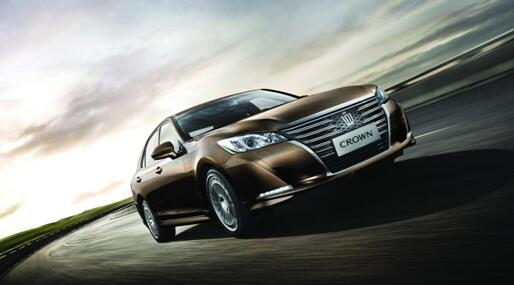从公布的全新CROWN皇冠2.5L行政版、智享版的价格和配置来看，一汽丰田为年轻新贵们准备了触手可得的“身份标签”，如果你有梦想，不要等以后，现在就去实现它。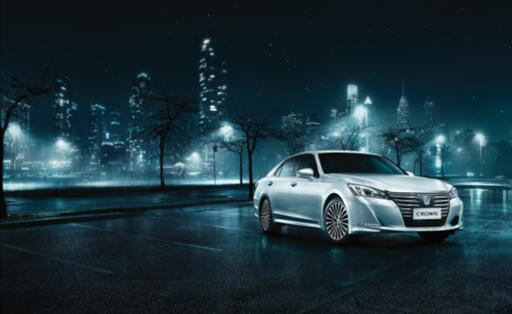价格为25.48万元的行政版，拥有全新CROWN皇冠豪华优雅的外观和尺寸，BOSS级的内部空间，并搭载了7英寸+5英寸TFT双屏可触多媒体显示系统、DSC起步辅助控制系统、紧急刹车警示系统以及智能弹起式发动机罩（保护行人）等诸多科技配置。配置如此丰富，价格却一再碰触年轻实力新贵的神经使一汽丰田整个产品阵营实现全面年轻化革新。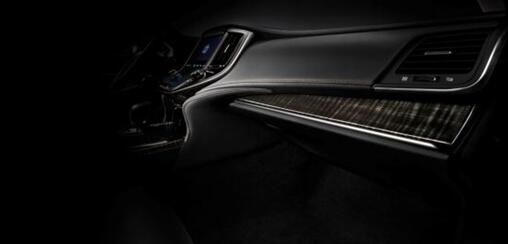智享版则配备了17英寸5辐轮毂、炬目LED组合式前大灯、木纹内饰装饰、真皮座椅、彩色信息显示屏、7英寸+5英寸TFT双屏可触多媒体显示系统、带杯架的后排中央扶手箱等，从视觉、触觉、满足感等多方面提升豪华品质，同时还有预碰撞安全系统、测距雷达（可视系统）等先进安全配置保驾护航。

除了超值的价格，以“挑战革新”为研发理念的全新CROWN皇冠，在传承品牌豪华理念的同时，实现了设计、动力以及安全方面的革新。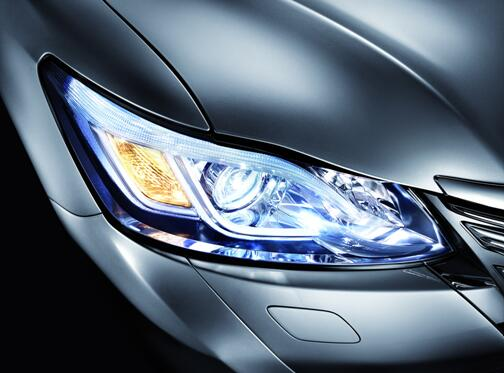全新CROWN皇冠“水平跃动”设计理念带来的动感外观，让人惊呼“有了脱胎换骨的改变”、“年轻了10岁”，昔日的中庸稳重印象一扫而光。在内饰上，全新CROWN皇冠也实现了“传承与革新的交融”，将尊贵感与科技感有机融为一体。首次搭载的TFT双屏可触多媒体显示系统依据人体工学简化了按钮设置，为消费者带来创新的外观感受和简洁的操作体验。另外，世界先进工艺打造的木纹饰件和精雕细琢而成的缝制饰件，营造出充满人性化关怀的舒适驾乘空间。值得称道的还是全新CROWN皇冠在动力方面的改变，拥有2.5L自然吸气+6AT和D-4ST 2.0T+8AT两种动力组合。2.0T+车型，成就了“科技+、动力+、舒适+、可靠+”的美名。搭载的发动机应用了四双【双喷射、双涡管、双循环、双VVT-i(W)】等技术，全面领先同级。更为难得的是，通过技术革新，2.0T+车型成功实现了规避机油浪费、延迟机油老化等目标，使其保持在正常消耗的范围内。同时，2.0T+车型搭载的手自一体式8档自动变速箱，专门匹配前置后驱车型。为了提升起步时的加速性能，全新研发的变矩器不仅减少了发动机震动，扩大锁止范围，还进一步提升了燃油经济性。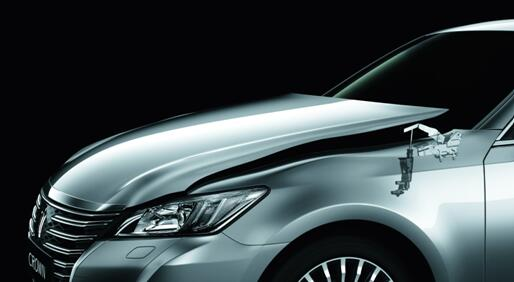安全方面全新CROWN皇冠依旧是行业标杆，全车系标配起步辅助控制系统DSC和智能弹起式发动机罩（保护行人）、SRS空气囊，高配车型更有车辆动态综合管理系统VDIM、预碰撞安全系统PCS、并线盲点监视器BSM、全景监控系统PVM等配置，成就了全新CROWN皇冠的顶级安全呵护。在权威的C-NCAP碰撞测试中，全新CROWN皇冠表现出对后排乘员的绝佳保护。三项测试中后排乘员共获得5.97分，得分率达到99.5%，在最难得分的正面40%碰撞试验中获得2分的满分，无论是总分还是每个详细考核项，均优于测试过的124个车型。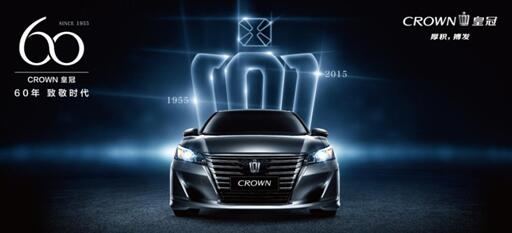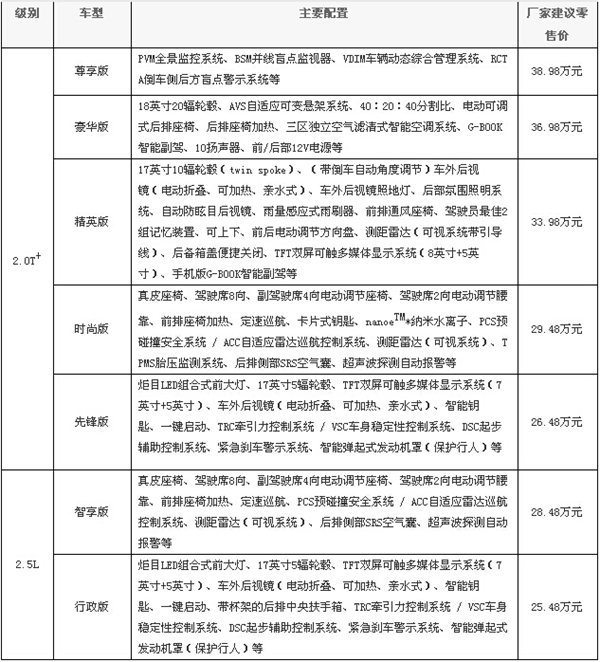承载着丰田60年的荣耀积淀和匠心之道，全新CROWN皇冠集合了全球领先的造车工艺和先进技术，无论是卓越的品质、领先的技术，还是拨乱反正的价格，都展现出领先时代、挑战变革的气魄。追逐梦想，创造未来的路上，全新CROWN皇冠一直会等你。

相关文章

﻿
• 快速找车
• 选择品牌
• 选择品牌
• A  奥迪
• A  阿斯顿·马丁
• A  阿尔法·罗密欧
• B  宝沃
• B  布加迪
• B  巴博斯
• B  保时捷
• B  宾利
• B  奔驰
• B  宝马
• B  本田
• B  别克
• B  标致
• B  比亚迪
• B  宝骏
• B  北汽制造
• B  北汽新能源
• B  北汽幻速
• B  北汽威旺
• B  北京汽车
• B  奔腾
• B  北汽绅宝
• B  北汽昌河
• C  长安欧尚
• C  长安
• C  长安凯程
• C  长城
• D  大众
• D  道奇
• D  DS
• D  东南
• D  东风风神
• D  东风风行
• D  东风小康
• D  东风风度
• D  东风
• F  福特
• F  丰田
• F  菲亚特
• F  法拉利
• F  福田
• F  福迪
• F  福汽启腾
• G  观致
• G  广汽传祺
• G  广汽吉奥
• G  GMC
• H  红旗
• H  汉腾汽车
• H  哈弗
• H  哈飞
• H  海格
• H  海马
• H  华颂
• H  黄海
• H  华泰
• H  恒天
• J  捷途
• J  几何汽车
• J  捷达
• J  吉利汽车
• J  捷豹
• J  Jeep
• J  江淮
• J  江铃
• J  金杯
• J  九龙
• J  金旅
• K  凯翼
• K  凯迪拉克
• K  克莱斯勒
• K  科尼塞克
• K  卡威
• K  开瑞
• L  路虎
• L  林肯
• L  劳斯莱斯
• L  兰博基尼
• L  雷克萨斯
• L  铃木
• L  领克
• L  雷诺
• L  理念
• L  力帆
• L  莲花汽车
• L  猎豹
• L  路特斯
• L  陆风
• M  马自达
• M  MG
• M  MINI
• M  玛莎拉蒂
• M  摩根
• M  迈凯轮
• N  纳智捷
• O  欧拉
• O  欧宝
• O  讴歌
• O  欧朗
• Q  奇瑞
• Q  起亚
• Q  启辰
• R  日产
• R  荣威
• R  瑞麒
• S  SERES赛力斯
• S  三菱
• S  斯威汽车
• S  萨博
• S  smart
• S  斯柯达
• S  斯巴鲁
• S  思铭
• S  双龙
• S  上汽大通
• S  双环
• T  特斯拉
• T  腾势
• W  蔚来
• W  沃尔沃
• W  WEY
• W  五菱汽车
• W  五十铃
• W  威兹曼
• W  威麟
• X  现代
• X  雪佛兰
• X  星途
• X  雪铁龙
• X  小鹏汽车
• X  西雅特
• Y  一汽
• Y  英菲尼迪
• Y  英致
• Y  依维柯
• Y  野马汽车
• Y  永源
• Z  众泰
• Z  中华
• Z  中兴
• Z  知豆
• 选择车系
• 选择车系
• 车型对比
• 选择品牌
• 选择品牌
• A  奥迪
• A  阿斯顿·马丁
• A  阿尔法·罗密欧
• B  宝沃
• B  布加迪
• B  巴博斯
• B  保时捷
• B  宾利
• B  奔驰
• B  宝马
• B  本田
• B  别克
• B  标致
• B  比亚迪
• B  宝骏
• B  北汽制造
• B  北汽新能源
• B  北汽幻速
• B  北汽威旺
• B  北京汽车
• B  奔腾
• B  北汽绅宝
• B  北汽昌河
• C  长安欧尚
• C  长安
• C  长安凯程
• C  长城
• D  大众
• D  道奇
• D  DS
• D  东南
• D  东风风神
• D  东风风行
• D  东风小康
• D  东风风度
• D  东风
• F  福特
• F  丰田
• F  菲亚特
• F  法拉利
• F  福田
• F  福迪
• F  福汽启腾
• G  观致
• G  广汽传祺
• G  广汽吉奥
• G  GMC
• H  红旗
• H  汉腾汽车
• H  哈弗
• H  哈飞
• H  海格
• H  海马
• H  华颂
• H  黄海
• H  华泰
• H  恒天
• J  捷途
• J  几何汽车
• J  捷达
• J  吉利汽车
• J  捷豹
• J  Jeep
• J  江淮
• J  江铃
• J  金杯
• J  九龙
• J  金旅
• K  凯翼
• K  凯迪拉克
• K  克莱斯勒
• K  科尼塞克
• K  卡威
• K  开瑞
• L  路虎
• L  林肯
• L  劳斯莱斯
• L  兰博基尼
• L  雷克萨斯
• L  铃木
• L  领克
• L  雷诺
• L  理念
• L  力帆
• L  莲花汽车
• L  猎豹
• L  路特斯
• L  陆风
• M  马自达
• M  MG
• M  MINI
• M  玛莎拉蒂
• M  摩根
• M  迈凯轮
• N  纳智捷
• O  欧拉
• O  欧宝
• O  讴歌
• O  欧朗
• Q  奇瑞
• Q  起亚
• Q  启辰
• R  日产
• R  荣威
• R  瑞麒
• S  SERES赛力斯
• S  三菱
• S  斯威汽车
• S  萨博
• S  smart
• S  斯柯达
• S  斯巴鲁
• S  思铭
• S  双龙
• S  上汽大通
• S  双环
• T  特斯拉
• T  腾势
• W  蔚来
• W  沃尔沃
• W  WEY
• W  五菱汽车
• W  五十铃
• W  威兹曼
• W  威麟
• X  现代
• X  雪佛兰
• X  星途
• X  雪铁龙
• X  小鹏汽车
• X  西雅特
• Y  一汽
• Y  英菲尼迪
• Y  英致
• Y  依维柯
• Y  野马汽车
• Y  永源
• Z  众泰
• Z  中华
• Z  中兴
• Z  知豆
• 选择车系
• 选择车系
• 选择车型
• 选择车型
• 意见反馈